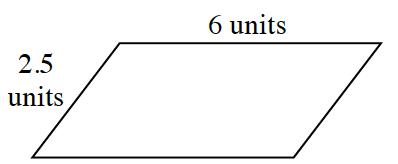### Home > CC2 > Chapter 6 > Lesson 6.2.3 > Problem6-89

6-89.Sketch the parallelogram shown at right, and then redraw it with sides that are half as long.

1. Find the perimeters of both the original and smaller parallelograms.

Label all four sides. The perimeter is the sum of all four lengths.

For the scaled figure, divide each side in half. Add all four of the half lengths.

2. If the height of the original parallelogram (drawn to the side that is $6$ units) is $2$ units, find the areas of both parallelograms.

Remember, the area of a parallelogram is $(\text{base})(\text{height})$.

For the smaller figure, would the area be half as much or a quarter as much of the larger figure? Drag the slider in the eTool below for a visual image.

Explore the problem with the eTool below.
Click the link at right for the full version of the eTool: 6-89 HW eTool (Desmos)